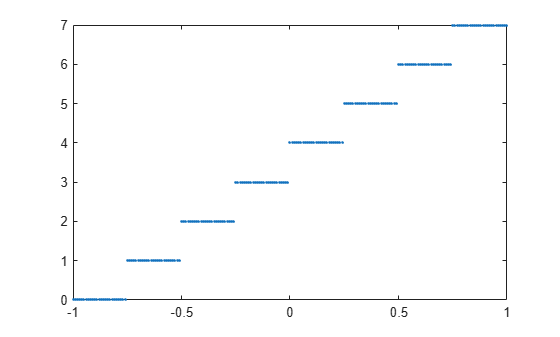# uencode

Quantize and encode floating-point inputs to integer outputs

## Syntax

``y = uencode(u,n)``
``y = uencode(u,n,v)``
``y = uencode(u,n,v,'SignFlag')``

## Description

example

````y = uencode(u,n)` quantizes the entries in a multidimensional array of floating-point numbers `u` and encodes them as integers using 2n-level quantization. The output y and the input `u` are arrays of the same size. The elements of the output y are unsigned integers with magnitudes in the range [0, 2n-1].```
````y = uencode(u,n,v)` allows the input `u` to have entries with floating-point values in the range `[-v,v]` before saturating them (the default value for `v` is 1).```
````y = uencode(u,n,v,'SignFlag')` maps entries in a multidimensional array of floating-point numbers `u` whose entries have values in the range [`-v,v`] to an integer output `y`. Input entries outside this range are saturated.```

## Examples

collapse all

Map floating-point scalars in [-1, 1] to `uint8` (unsigned) integers. Produce a staircase plot. The horizontal axis ranges from -1 to 1 and the vertical axis from 0 to 7 (i.e., ${2}^{3}-1$).

```u = -1:0.01:1; y = uencode(u,3); plot(u,y,'.')```Look at saturation effects when you underspecify the peak value for the input.

```u = -2:0.5:2; y = uencode(u,5,1)```
```y = 1x9 uint8 row vector 0 0 0 8 16 24 31 31 31 ```

Specify you want signed output.

```u = -2:0.5:2; y = uencode(u,5,2,'signed')```
```y = 1x9 int8 row vector -16 -12 -8 -4 0 4 8 12 15 ```

## Input Arguments

collapse all

Floating point input, specified as a matrix or a vector. The input may be real or complex. Elements of the input `u` outside of the range `[-1,1]` are treated as overflows and are saturated as:

• For entries in the input `u` that are less than -1, the value of the output of `uencode` is 0.

• For entries in the input `u` that are greater than 1, the value of the output of `uencode` is 2n-1.

Data Types: `single` | `double`

Measure of number of quantization levels, specified as a positive integer scalar. `n` must be an integer between 2 and 32 (inclusive).

Peak value, specified as a positive real scalar. Elements of `u` outside of the range `[-v,v]` are treated as overflows and are saturated:

• For input entries less than -`v`, the value of the output of `uencode` is 0.

• For input entries greater than `v`, the value of the output of `uencode` is 2n – 1.

Sign of output, specified as `'signed'` or `'unsigned'`. The integer type of the output depends on the number of quantization levels 2n and the value of `'SignFlag'`, which can be one of the following:

• `'signed'`: Outputs are signed integers with magnitudes in the range [-2n/2, (2n/2) – 1].

• `'unsigned'` (default): Outputs are unsigned integers with magnitudes in the range [0, 2n – 1].

## Output Arguments

collapse all

Encoded integer outputs, returned as a vector or a matrix.

## Algorithms

`uencode` maps the floating-point input value to an integer value determined by the requirement for 2n levels of quantization. This encoding adheres to the definition for uniform encoding specified in ITU-T Recommendation G.701. The input range `[-v,v]` is divided into 2n evenly spaced intervals. Input entries in the range `[-v,v]` are first quantized according to this subdivision of the input range, and then mapped to one of 2n integers. The range of the output depends on whether or not you specify that you want signed integers.

The output data types are optimized for the number of bits as shown in the table below.

 International Telecommunication Union. General Aspects of Digital Transmission Systems: Vocabulary of Digital Transmission and Multiplexing, and Pulse Code Modulation (PCM) Terms. ITU-T Recommendation G.701. March, 1993.